# 博客开始支持LaTeX啦

$$\LaTeX$$

$$\frac{a}{b}\pm \frac{c}{d}= \frac{ad \pm bc}{bd}$$

$$\ce{SO4^2- + Ba^2+ -> BaSO4 v}$$

$$\sin \alpha + \sin \beta =2 \sin \frac{\alpha + \beta}{2}\cos \frac{\alpha - \beta}{2}$$

$$\ce{Zn^2+ <=>[+ 2OH-][+ 2H+] \underset{\text{amphoteres Hydroxid}}{\ce{Zn(OH)2 v}} <=>[+ 2OH-][+ 2H+] \underset{\text{Hydroxozikat}}{\ce{[Zn(OH)4]^2-}}}$$


$\LaTeX$

$\frac{a}{b}\pm \frac{c}{d}= \frac{ad \pm bc}{bd}$

$\ce{SO4^2- + Ba^2+ -> BaSO4 v}$

$\sin \alpha + \sin \beta =2 \sin \frac{\alpha + \beta}{2}\cos \frac{\alpha - \beta}{2}$

\ce{Zn^2+ <=>[+ 2OH-][+ 2H+] $\underset{\text{amphoteres Hydroxid}}{\ce{Zn(OH)2 v}}$ <=>[+ 2OH-][+ 2H+] $\underset{\text{Hydroxozikat}}{\ce{[Zn(OH)4]^2-}}$}

mathjax: true


•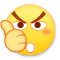0
•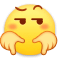0
•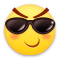0
•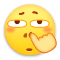0
•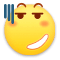0
•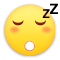0

• 按正序
• 按倒序
• 按热度